# Create Histogram in Excel  Step by Step Guide ▷ 2020

One of the advantages of using this calculation software is that you will have the opportunity to have a great number of tools and elements when working on it, which will guarantee a completely professional work, since you can have all the necessary functions so you can create the calculation document you want.

This is how you will have the opportunity to create a histogram, which will allow you sort all data by categories. Allowing you to show through a graph a frequency distribution of the data you are working with. It is important to remember that this is a graphical representation of variables in the form of bars.

Where each of you are bars is proportional to the frequency of the value it is representing. This is how here we are going to explain a little more about what is this element and how can you start using it in your Excel spreadsheet. To do this, follow in detail everything that we will explain in the post.

## What is a histogram and what is this graph for?

A histogram or as it is also known graph or diagram, is that representation that shows the number of times is that each of the values ​​that is being considered is repeated and is mainly used when handling successive measurements. In this way you can say that indicate the frequency of a fact through a data distribution.

It is important to clarify that this type of graphic representations they can’t create with attributes, but must be done through measurable variables such as numbers, temperature, times, weights, among some others. All this will allow us to see around what value are grouped measurements, as is the dispersion around the central value and the central tendency.

To end, the histogramss are nothing more than a one-variable bar graph, where each of the bars will be proportional to the frequency of the represented values. Himself has a x axis and y axis, usually in the X axis is the frequencies, Meanwhile in the Y axis the values ​​of the variables.

It is also important to mention that there are different types of histograms which we will show you below:

• Simple bar diagrams: These representations show the simple frequency by the height of the bar, which is proportional to the frequency of each of the values ​​they are representing.
• Composite bar diagram: It is used to represent two variables, where the height of the bar with which it is will represent the simple frequency of the modalities or categories of the variables and this height is proportional to the simple frequency of each one of the modalities.
• Clustered bar chart: It is used to represent the information of two variables, where it is represented through a set of bars that is classified with respect to the different modalities.
• Frequency polygon: It consists of a line graph that is used to represent absolute frequencies of the values ​​of a distribution. In this case the height is the point associated with a value of the variables and is proportional to the frequency of said value.
• Percentage warhead: It’s about a cumulative graph, which is very useful especially when you want to represent the percentage range of each of the values ​​within a frequency distribution.

## What are the best Excel tools to create a Histogram?

When you want create a histogram in Microsoft Excel it is important to be able activate tools for analysis, as this works as a plugin that will allow how this chart works correctly.

Therefore, the first thing you should do is the following:

### Activate tool for analysis

The first thing will be to activate this function, to do this follow the following steps:

• You must go to the tab “Archive” of your spreadsheet, and there you must select the item from “Options”.
• Then select “Complement” and subsequently “Excel Add-in”.
• The following window will appear on the screen where you must activate the option of “Tool for analysis”.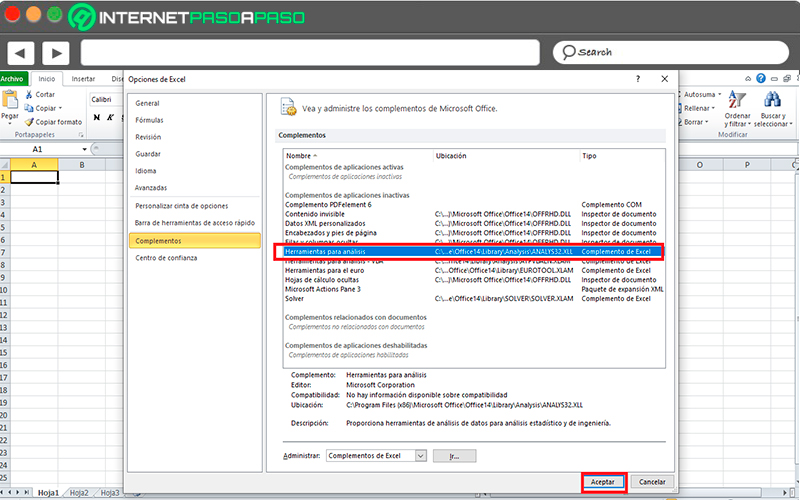• Once you have activated this tool for analysis, the next thing will be to go to the tab of “Data” and within the group of “Analysis” click on the command “Analysis of data” so that the following box appears.
• There you will select the option “Histogram”, this will allow you to specify the necessary arguments to be able to classify each of the data.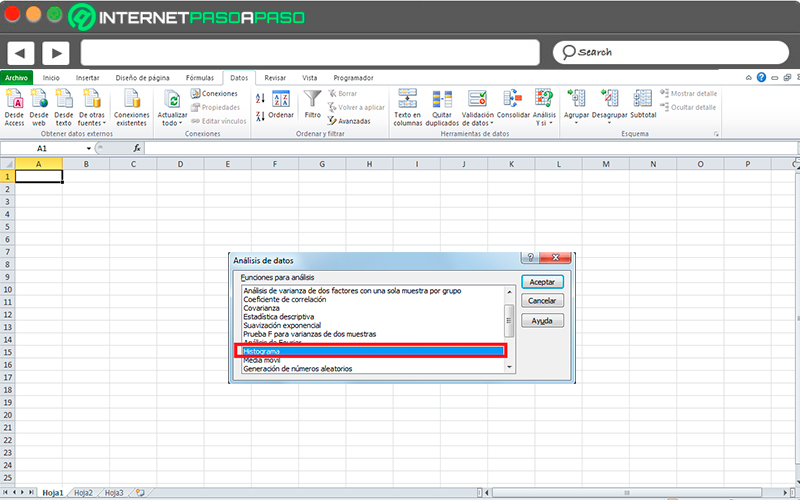• How is he going to do it “Input range”, here you must specify which range contains the data from your excel sheet, note that you should not include the Title of each column, but only the data that you want to be taken into account.
• In the box “Class range” You must insert the range of cells with the class previously created.
• You must also check the box of a “New leaf” and check the option of “Create chart” and finally click on “To accept”.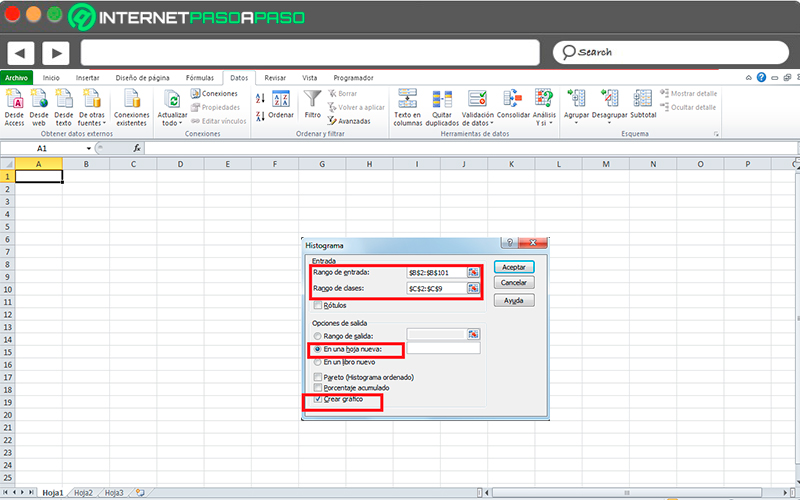• This way you will be applying the necessary Excel tools at the moment of wanting create a histogram on the spreadsheet.

## Steps to create a histogram in Microsoft Excel like a pro

Once everything mentioned above is completely clear, the following will be create a histogram in Microsoft Excel, it is important to mention that this procedure is quite simple to perform.

To do this, you must follow each of the steps that we are going to explain below:

• To build a histogram in Microsoft software it will be necessary to group all the data by classes, that is, by categories, since this will facilitate much more the work to be done.
• For this example we will use a group of people with different ages. In the case that you don’t know the classes of the input data then you can make a copy of data range and use the remove duplicates command, this will give you a list of unique values.
• In this case, the range of ages collected is known, which is between 18 and 25 years old, so that column C can be entered manually.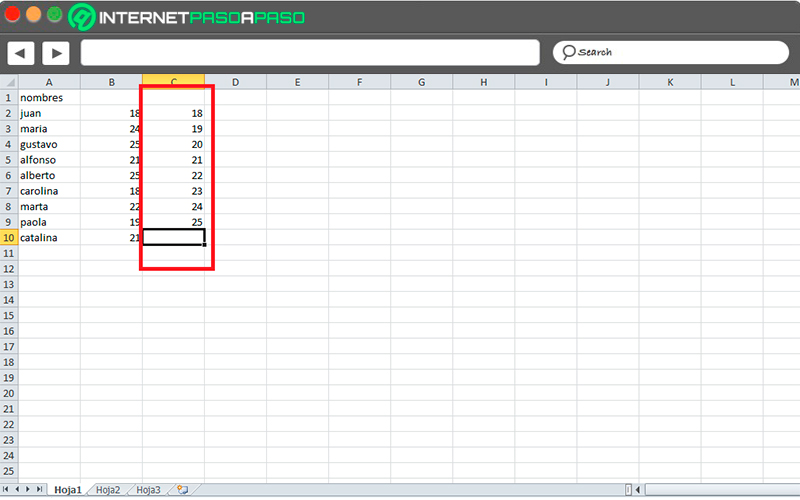• The following will be activate the tool for analysis, this you can do as it was explained in the previous section through the complements.
• After activating the analysis tool you must go to the analysis option and there select “Histogram”.
• The following will be mark histogram range that you want to create, in this case you must the input range as the class range. Once you have selected all this you must click on “To accept”, which will give you the following result: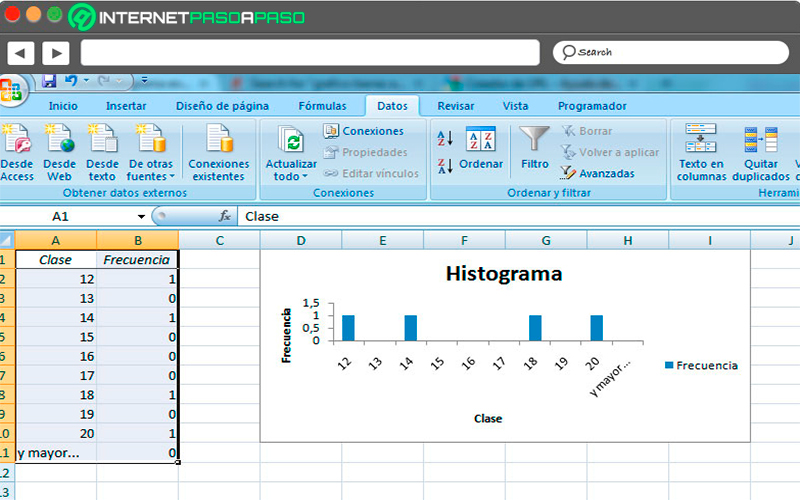If you have any questions, leave them in the comments, we will answer you as soon as possible, and it will surely be of great help to more members of the community. Thank you! 😉

Rate this post